# Solenoid

A long straight coil of wire can be used to generate a nearly uniform magnetic field similar to that of a bar magnet. Such coils, called solenoids, have an enormous number of practical applications. The field can be greatly strengthened by the addition of an iron core. Such cores are typical in electromagnets.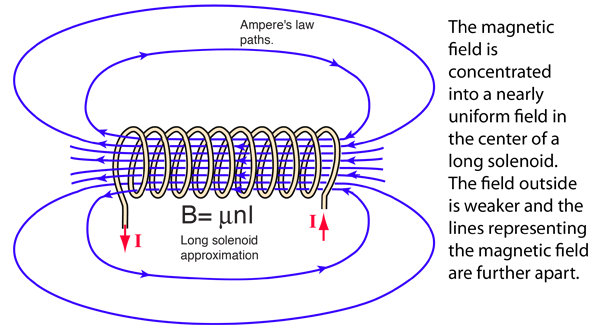In the above expression for the magnetic field B, n = N/L is the number of turns per unit length, sometimes called the "turns density". The magnetic field B is proportional to the current I in the coil. The expression is an idealization to an infinite length solenoid, but provides a good approximation to the field of a long solenoid.

 Derive field expression Calculate field Field of current loop
 The solenoid as an inductor Superconducting magnets
Index

Magnetic field concepts

Currents as magnetic sources

 HyperPhysics***** Electricity and Magnetism R Nave
Go Back

# Solenoid Field from Ampere's Law

Taking a rectangular path about which to evaluate Ampere's Law such that the length of the side parallel to the solenoid field is L gives a contribution BL inside the coil. The field is essentially perpendicular to the sides of the path, giving negligible contribution. If the end is taken so far from the coil that the field is negligible, then the length inside the coil is the dominant contribution.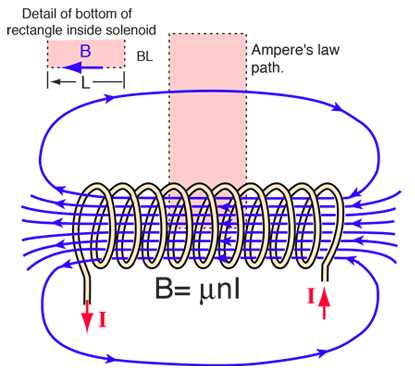This admittedly idealized case for Ampere's Law gives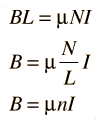This turns out to be a good approximation for the solenoid field, particularly in the case of an iron core solenoid.
 Solenoid discussion Calculate field
Index

Magnetic field concepts

Currents as magnetic sources

 HyperPhysics***** Electricity and Magnetism R Nave
Go Back

# Solenoid Magnetic Field Calculation

 At the center of a long solenoid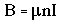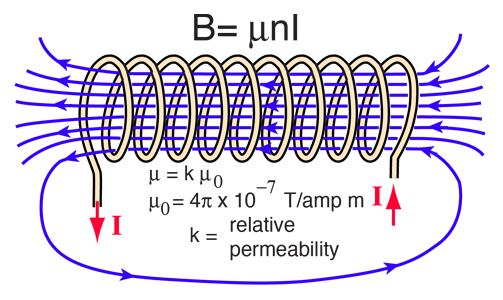Active formula: click on the quantity you wish to calculate. Magnetic field = permeability x turn density x current

For a solenoid of length L = m with N = turns,
the turn density is n=N/L= turns/m.

If the current in the solenoid is I = amperes

and the relative permeability of the core is k = ,

then the magnetic field at the center of the solenoid is

B = Tesla = gauss.

The Earth's magnetic field is about half a gauss.

The relative permeability of magnetic iron is around 200.

Enter data, then click on the quantity you wish to calculate in the active formula above the data entry points. Default values will be entered for unspecified parameters, but the numbers will not be forced to be consistent until you click on the quantity to calculate.

 Solenoid discussion Derive field expression Relative permeability
Index

Magnetic field concepts

Currents as magnetic sources

 HyperPhysics***** Electricity and Magnetism R Nave
Go Back